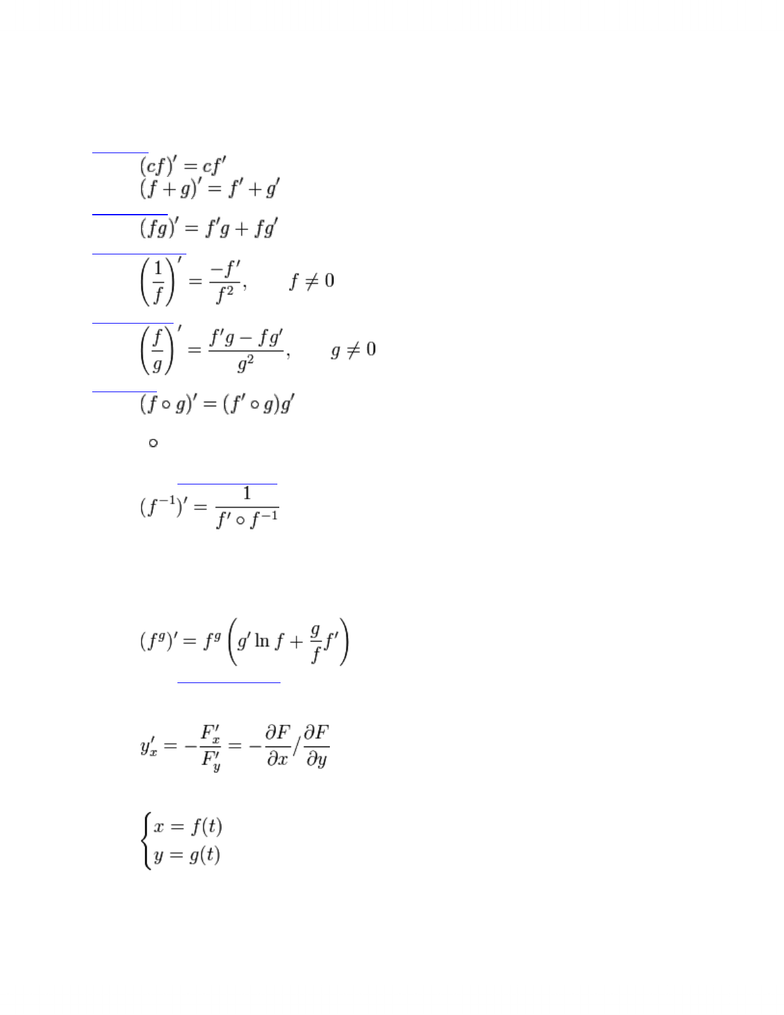# FIN200H1 Lecture Notes - Product Rule

49 views3 pages
School
Department
CourseGeneral differentiation rules
Linearity
Product rule
Reciprocal rule
Quotient rule
Chain rule
where (f g)(x) is defined as f(g(x))
Derivative of inverse function
for any differentiable function f of a real argument and with real values, when the indicated
compositions and inverses exist.
Generalized power rule
Derivative of implicit function
If implicit function y(x) is defined as F(x,y(x)) = 0
then
Derivative of parametrically defined function
If a function y(x) defined parametrically
then
Unlock document

This preview shows page 1 of the document.
Unlock all 3 pages and 3 million more documents.

# Get access

\$10 USD/m
Billed \$120 USD annually
Homework Help
Class Notes
Textbook Notes